Add Dotted Line for Future Data

This post will guide you how to add the dotted line for the forecasted data in an existing line chart in excel.

Assuming that you have a line chart that shows the actual data, and you can also add the forecast values as another data series, and how to add those newly forecast values into the existing line chart as a dotted line.

Add Dotted Line for Future Data

To add the dotted line for the forecasted data in one excel chart, just do the following steps:

#1 insert one column and add the forested data into this column.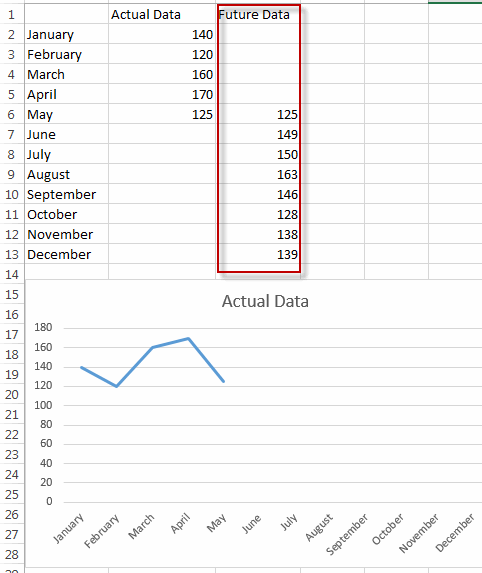#2 select the current line chart, right click on it, and select Select Data… from the pop-up menu list. The Select Data Source dialog will appear.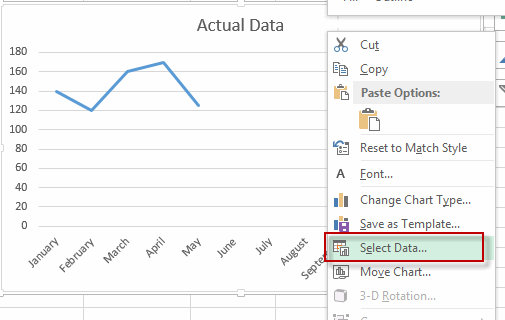#3 click Add button under Legend Entries section. The Edit Series dialog will appear.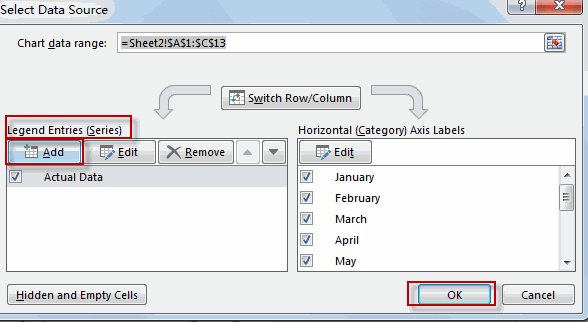#4 type one series name in the Series name text box, then select the data in the future data column. Click OK.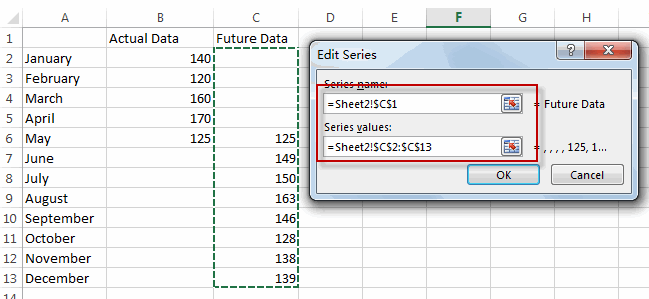#5 you will see that the line of the future data is added in the existing chart.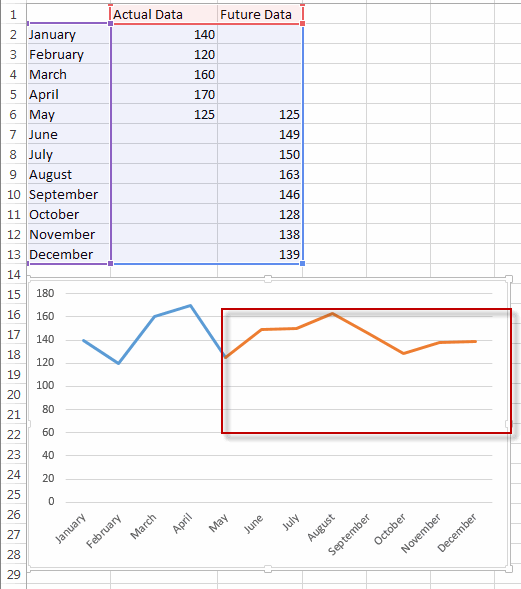#6 select the newly added line, right click on it, and select Format Data Series… menu from the pop up menu list. The Format Data Series pane is shown in the right of window.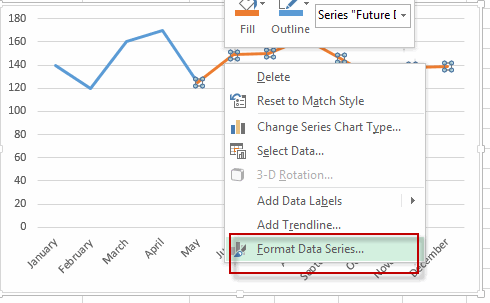#7 click Fill&Line, select Square Dot from the Dash type drop-down list.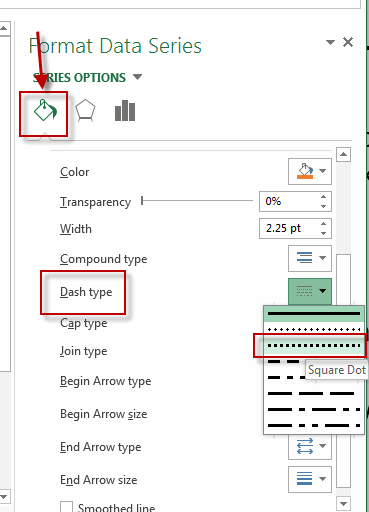#8 let’s see the result.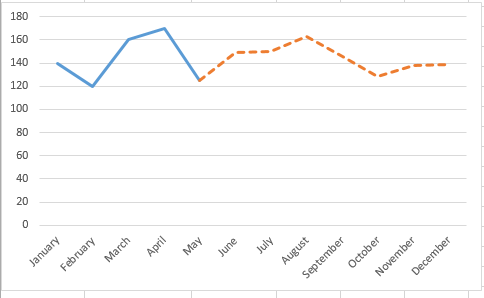Related Posts

Filter Data by Column and Sort by Row

This post will guide you how to use the FILTER function to filter a value by column and then sort data by row in Microsoft Excel. You can use the following formula based on the SORT function in combination with ...

Filter And Transpose Data From Horizontal To Vertical

This post will show you how to use Filter function and in combination with Transpose function to filter data from horizontal and transpose data as vertical in Microsoft Excel. You can refer to the below general formula based on TRANSPOSE ...

VLOOKUP Formula | Faster Trick with 2 VLOOKUPS

This post will guide you how to use 2 VLOOKUPS function to looking up data entries from a given range of cells in Microsoft Excel. VLOOKUP with 2 lookups can be faster than a single VLOOKUP in certain scenarios. The ...

Extract Unique Items From A List

This post will guide you how to extract unique itmes from a given list in Microsoft Excel. How to create a newly formula to get unique values from a range cells in Excel. The unique list of items is the ...

Extract substring In Excel

This post will guide you how to use Excel's MID function is a quick and easy way to extract pieces from your text. Use the Excel formula to extract a substring with MID. Note: If you want to extract just ...

How to Use 3D SUM Multiple Worksheets

To sum a range of numbers is straightforward for most Excel users, but do you know how to establish a 3D reference to total the same range of numerous sheets. In this post, I will present the steps for this ...

Extract all the matches with helper Column

With Excel's powerful functions IF, INDEX, and MATCH, we can find exactly what you're looking for with a few clicks of the mouse. This step-by-step tutorial will show how easy it is to extract data using these tools and more! ...

If Cell is This Value or That Value

IF function is frequently used in Excel worksheet to return you expect “true value” or “false value” based on the result of logical test. If you want to see if a cell is A or B, and if one of ...

If Value is Greater Than A Certain Value

IF function is frequently used in Excel worksheet to return you expect “true value” or “false value” based on the logical test result. If you want to see if a value in one cell is greater than a specific value, ...

If Cell is Not Blank

IF function is frequently used in Excel worksheet to return you expect “true value” or “false value” based on the result of created logical test. If you want to see if a cell is blank or not, and leave some ...

Sidebar